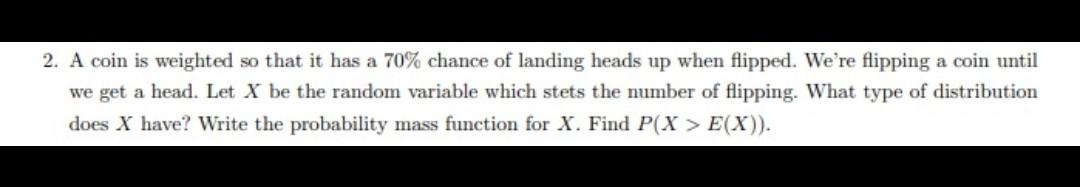# Question Solved1 Answer2. A coin is weighted so that it has a 70% chance of landing heads up when flipped. We're flipping a coin until we get a head. Let X be the random variable which stets the number of flipping. What type of distribution does X have? Write the probability mass function for X. Find P(X > E(X)).EKB31F The Asker · Probability and StatisticsTranscribed Image Text: 2. A coin is weighted so that it has a 70% chance of landing heads up when flipped. We're flipping a coin until we get a head. Let X be the random variable which stets the number of flipping. What type of distribution does X have? Write the probability mass function for X. Find P(X > E(X)).
More
Transcribed Image Text: 2. A coin is weighted so that it has a 70% chance of landing heads up when flipped. We're flipping a coin until we get a head. Let X be the random variable which stets the number of flipping. What type of distribution does X have? Write the probability mass function for X. Find P(X > E(X)).